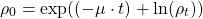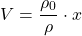# Seeding volumens

It is important to split or harvest a cell culture at a defined cell density. Depending on the cell line splitting at the wrong cell density can have many negative effects. You can observe e.g. a lower growth rate, problems with detaching of adherent cells, formation of aggregates, reduced cell viability, irreversible changes like beginning of differentiation processes, changes in receptor expression and many more.

You can use this tool to find the correct seeding density of your cells for a defined period (e.g. 2, 3, 4, … days).

You just need the growth rate (see growth rate calculator) and optimal harvest density of your cells.

You would like to know how the values are calculated? –  Have a look at the equations.

ρ = cell density [cells/ cm2]

ρ0= seeding density [cells/ cm2]

ρt = planned harvest density [cells/ cm2]

μ = growth rate [1/d]

t = days until next passage

V = volume of suspension per vessel [mL]

x = size of culture vessel [cm2]

N v = number of vessels